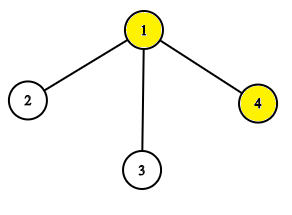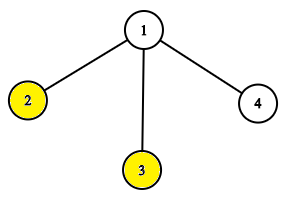# CF877E Danil and a Part-time Job

• 46通过
• 102提交
• 题目来源
• 评测方式 RemoteJudge
• 标签
• 难度 省选/NOI-
• 时空限制 2000ms / 256MB
• 提示：收藏到任务计划后，可在首页查看。

## 题意翻译

题目大意:

有一棵 $n$ 个点的树，根结点为 $1$ 号点，每个点的权值都是 $1$ 或 $0$

共有 $m$ 次操作，操作分为两种

$get$ 询问一个点 $x$ 的子树里有多少个 $1$

$pow$ 将一个点 $x$ 的子树中所有节点取反

对于每个 $get$ 给出答案

输入格式：

第一行一个整数 $n$

第二行共 $n-1$ 个整数，第 $i$ 个数 $x_i$ 表示 $x_i$ 是 $i+1$ 的父亲，

第三行给出每个点的初始权值

第四行一个整数 $m$

接下来 $m$ 行为操作类型和位置

## 题目描述

Danil decided to earn some money, so he had found a part-time job. The interview have went well, so now he is a light switcher.

Danil works in a rooted tree (undirected connected acyclic graph) with $n$ vertices, vertex $1$ is the root of the tree. There is a room in each vertex, light can be switched on or off in each room. Danil's duties include switching light in all rooms of the subtree of the vertex. It means that if light is switched on in some room of the subtree, he should switch it off. Otherwise, he should switch it on.

Unfortunately (or fortunately), Danil is very lazy. He knows that his boss is not going to personally check the work. Instead, he will send Danil tasks using Workforces personal messages.

There are two types of tasks:

1. pow v describes a task to switch lights in the subtree of vertex $v$ .
2. get v describes a task to count the number of rooms in the subtree of $v$ , in which the light is turned on. Danil should send the answer to his boss using Workforces messages.

A subtree of vertex $v$ is a set of vertices for which the shortest path from them to the root passes through $v$ . In particular, the vertex $v$ is in the subtree of $v$ .

Danil is not going to perform his duties. He asks you to write a program, which answers the boss instead of him.

## 输入输出格式

输入格式：

The first line contains a single integer $n$ ( $1<=n<=200000$ ) — the number of vertices in the tree.

The second line contains $n-1$ space-separated integers $p_{2},p_{3},...,p_{n}$ ( $1<=p_{i}&lt;i$ ), where $p_{i}$ is the ancestor of vertex $i$ .

The third line contains $n$ space-separated integers $t_{1},t_{2},...,t_{n}$ ( $0<=t_{i}<=1$ ), where $t_{i}$ is $1$ , if the light is turned on in vertex $i$ and $0$ otherwise.

The fourth line contains a single integer $q$ ( $1<=q<=200000$ ) — the number of tasks.

The next $q$ lines are get v or pow v ( $1<=v<=n$ ) — the tasks described above.

输出格式：

For each task get v print the number of rooms in the subtree of $v$ , in which the light is turned on.

## 输入输出样例

输入样例#1： 复制
4
1 1 1
1 0 0 1
9
get 1
get 2
get 3
get 4
pow 1
get 1
get 2
get 3
get 4

输出样例#1： 复制
2
0
0
1
2
1
1
0


## 说明The tree before the task pow 1.The tree after the task pow 1.

提示
标程仅供做题后或实在无思路时参考。
请自觉、自律地使用该功能并请对自己的学习负责。
如果发现恶意抄袭标程，将按照I类违反进行处理。